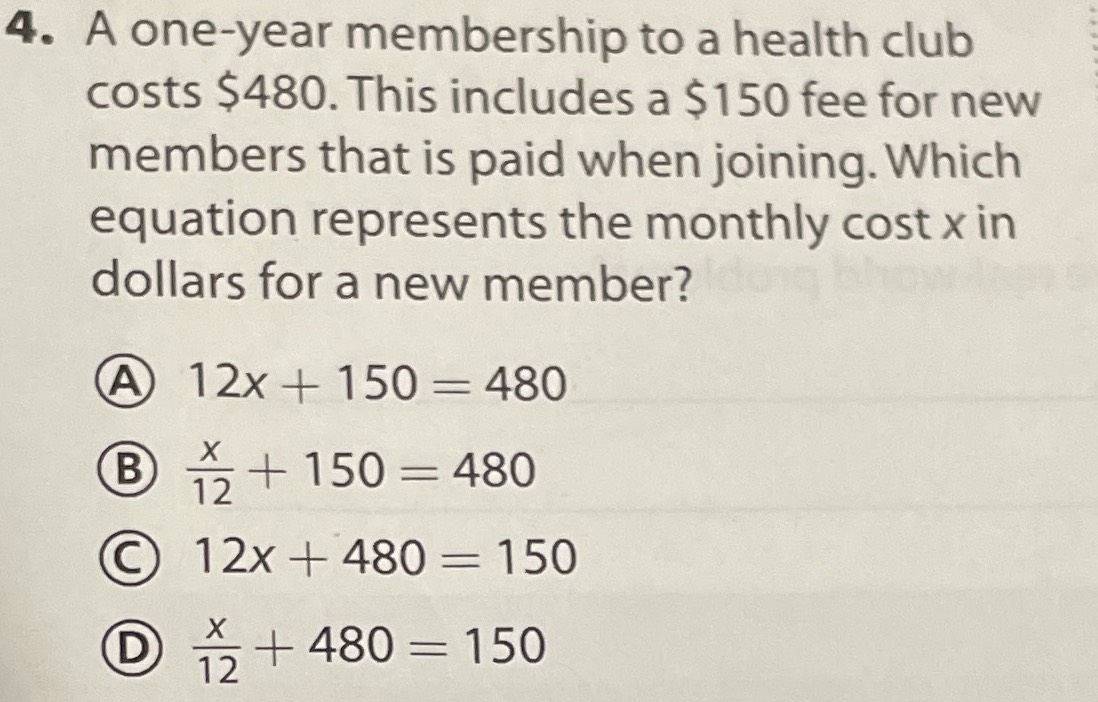### ¿Todavía tienes preguntas de matemáticas?

Pregunte a nuestros tutores expertos
Algebra
Pregunta4. A one-year membership to a health club costs $$\ 480$$ . This includes a $$\ 150$$ fee for new members that is paid when joining. Which equation represents the monthly cost $$x$$ in dollars for a new member?

(A) $$12 x + 150 = 480$$

(B) $$\frac { x } { 12 } + 150 = 480$$

(C) $$12 x + 480 = 150$$

(D) $$\frac { x } { 12 } + 480 = 150$$